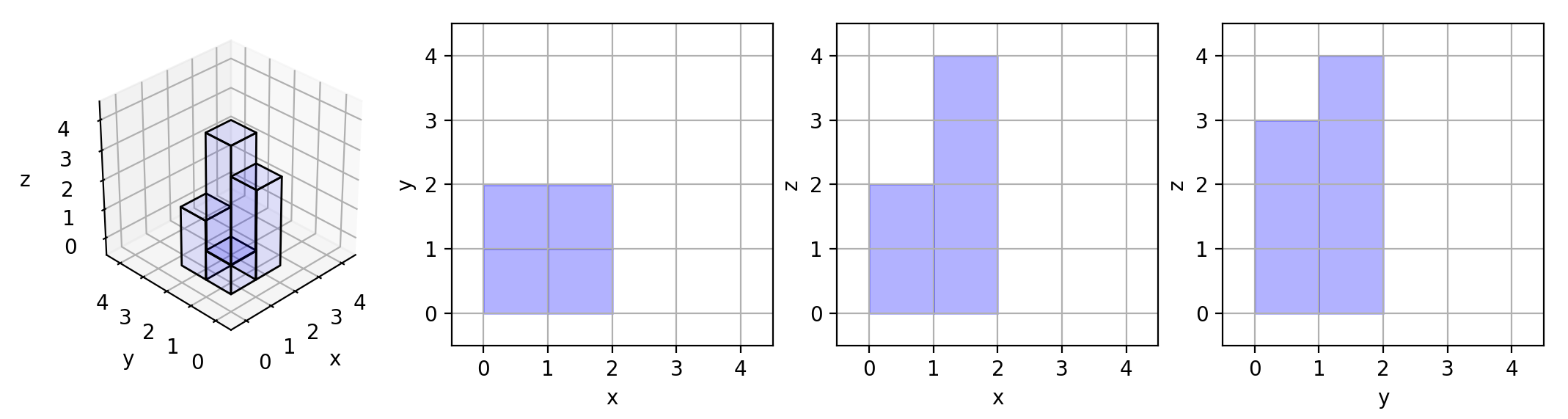# 883.projection area of 3d shapes## Description

On a N N grid, we place some 1 1 * 1 cubes that are axis-aligned with the x, y, and z axes.

Each value v = grid[i][j] represents a tower of v cubes placed on top of grid cell (i, j).

Now we view the projection of these cubes onto the xy, yz, and zx planes.

A projection is like a shadow, that maps our 3 dimensional figure to a 2 dimensional plane.

Here, we are viewing the “shadow” when looking at the cubes from the top, the front, and the side.

Return the total area of all three projections.

### Example 1:

``````Input: []
Output: 5
``````

### Example 2:

``````Input: [[1,2],[3,4]]
Output: 17
Explanation:
Here are the three projections ("shadows") of the shape made with each axis-aligned plane.
``````### Example 3:

``````Input: [[1,0],[0,2]]
Output: 8
``````

### Example 4:

``````Input: [[1,1,1],[1,0,1],[1,1,1]]
Output: 14
``````

### Example 5:

``````Input: [[2,2,2],[2,1,2],[2,2,2]]
Output: 21
``````

### Note:

• 1 <= grid.length = grid.length <= 50
• 0 <= grid[i][j] <= 50

1. updating

## 参考代码

``````class Solution:
def projectionArea(self, grid):
row=len(grid)
col=len(grid)
index=0
for i in range(row):
index+=max(grid[i])
for j in range(col):
ii=0
for i in range(row):
if(grid[i][j]!=0):
index+=1
ii=max(ii,grid[i][j])
index+=ii
return index
``````